Скачать презентацию Price discrimination A producer is able to charge

166b078a96e53409aae59b1f6490de68.ppt

• Количество слайдов: 39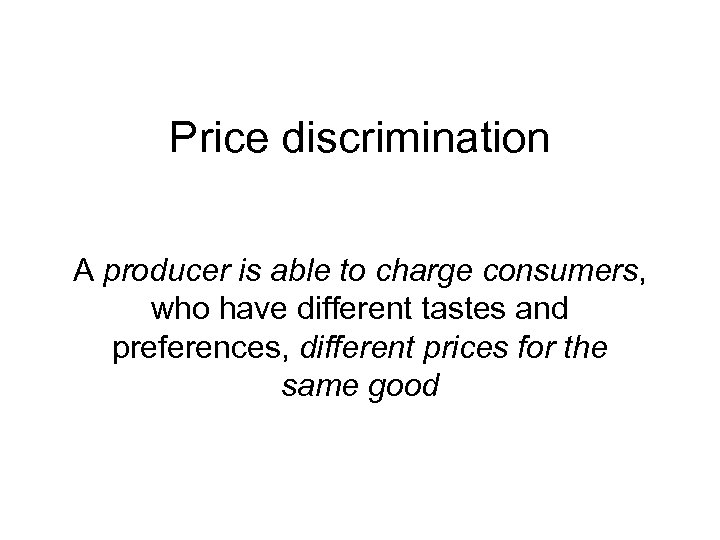Price discrimination A producer is able to charge consumers, who have different tastes and preferences, different prices for the same good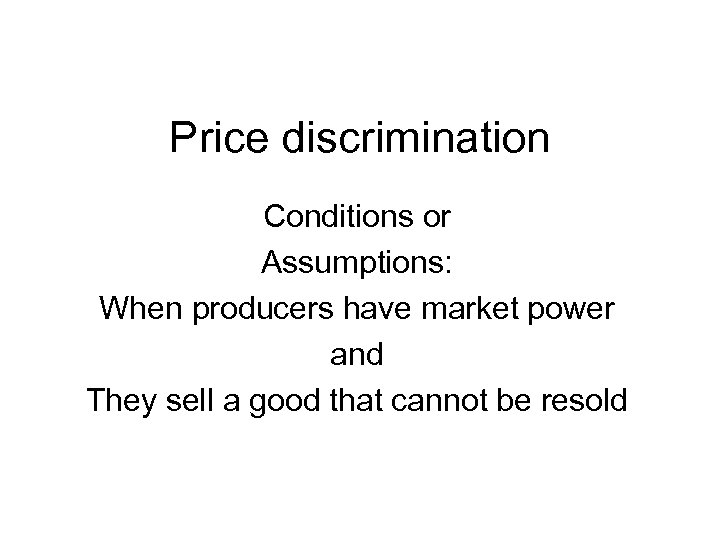Price discrimination Conditions or Assumptions: When producers have market power and They sell a good that cannot be resold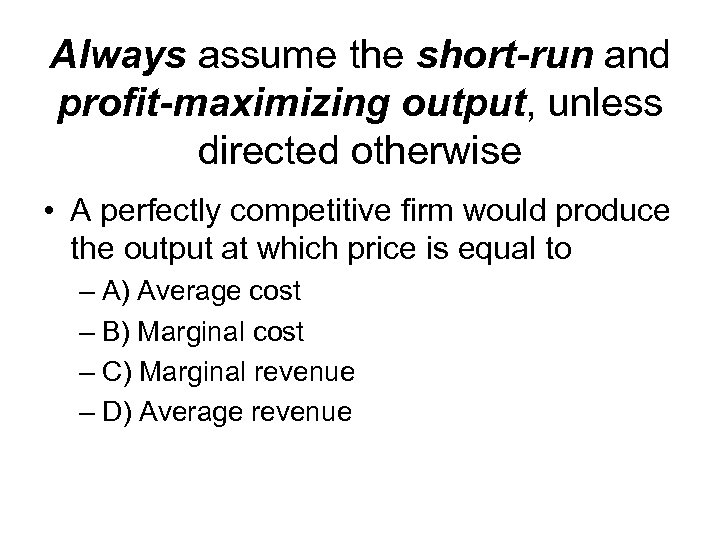Always assume the short-run and profit-maximizing output, unless directed otherwise • A perfectly competitive firm would produce the output at which price is equal to – A) Average cost – B) Marginal cost – C) Marginal revenue – D) Average revenue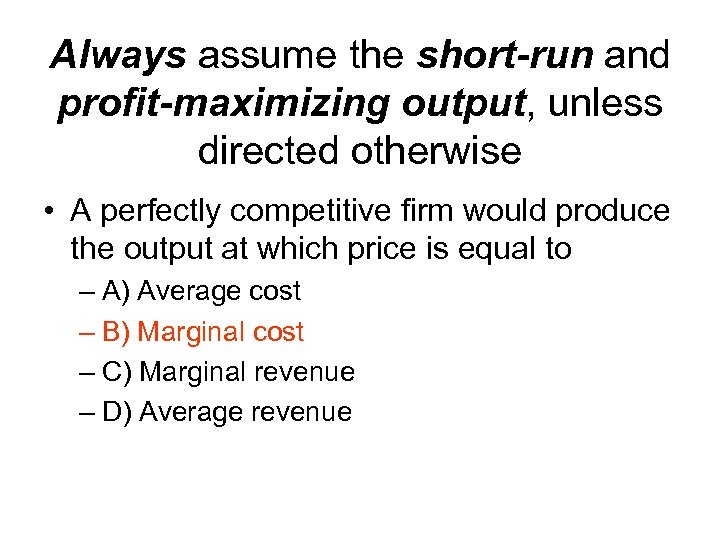Always assume the short-run and profit-maximizing output, unless directed otherwise • A perfectly competitive firm would produce the output at which price is equal to – A) Average cost – B) Marginal cost – C) Marginal revenue – D) Average revenue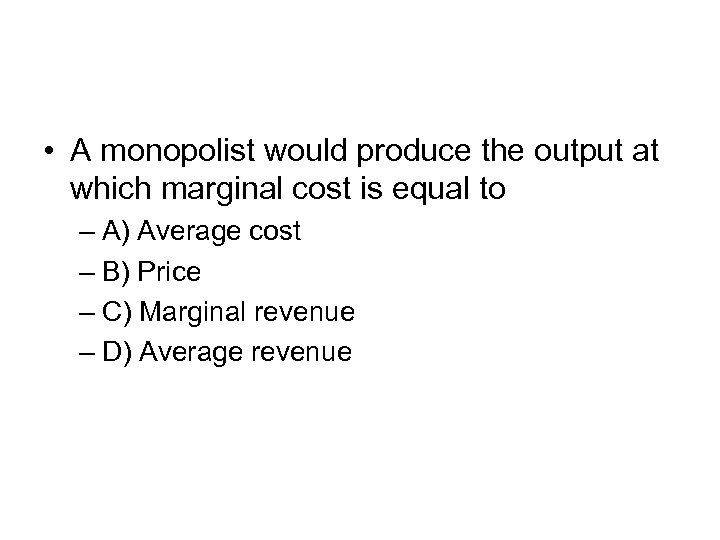• A monopolist would produce the output at which marginal cost is equal to – A) Average cost – B) Price – C) Marginal revenue – D) Average revenue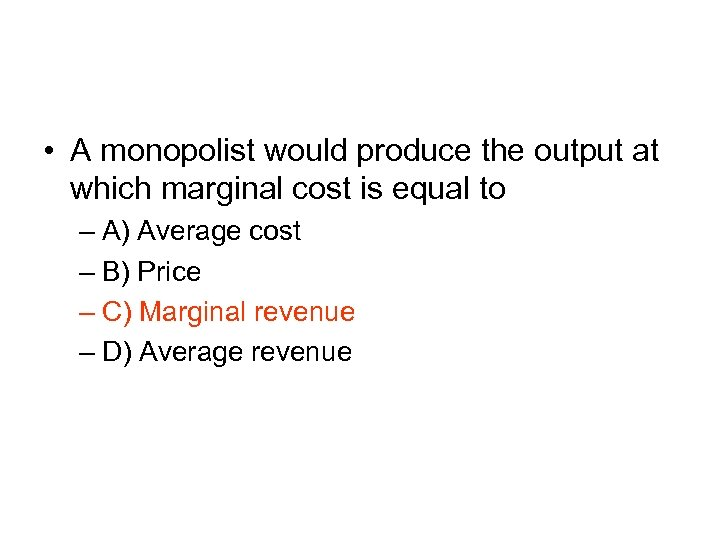• A monopolist would produce the output at which marginal cost is equal to – A) Average cost – B) Price – C) Marginal revenue – D) Average revenue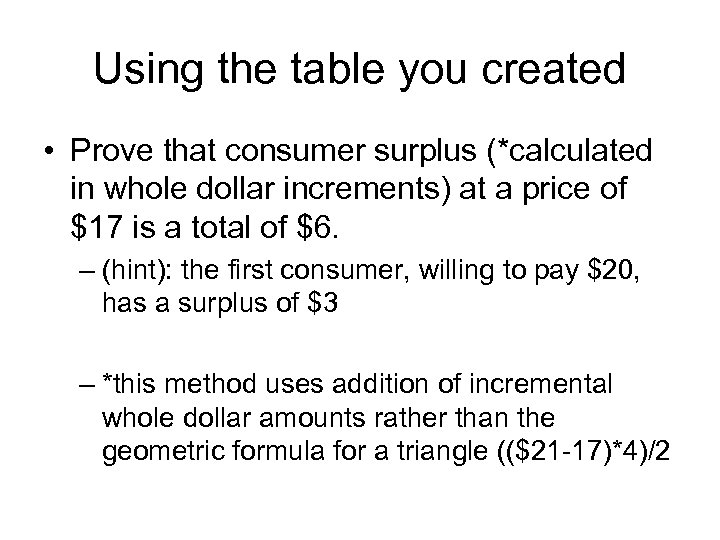Using the table you created • Prove that consumer surplus (*calculated in whole dollar increments) at a price of \$17 is a total of \$6. – (hint): the first consumer, willing to pay \$20, has a surplus of \$3 – *this method uses addition of incremental whole dollar amounts rather than the geometric formula for a triangle ((\$21 -17)*4)/2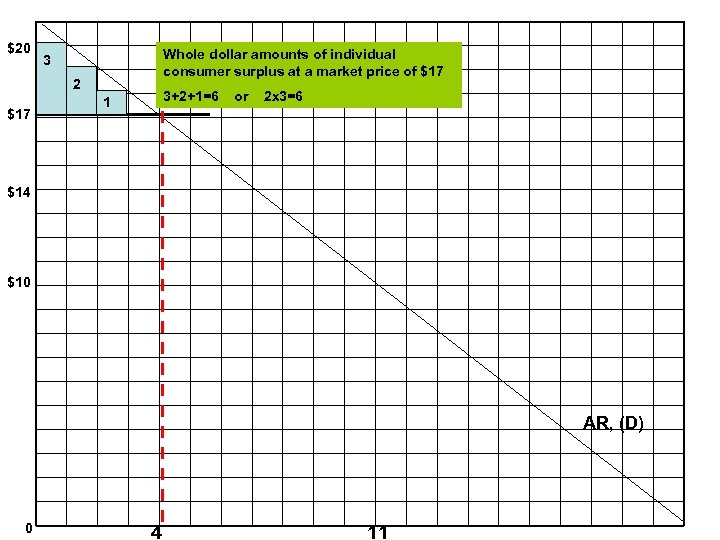\$20 Whole dollar amounts of individual consumer surplus at a market price of \$17 3 2 \$17 3+2+1=6 1 or 2 x 3=6 \$14 \$10 AR, (D) 0 4 11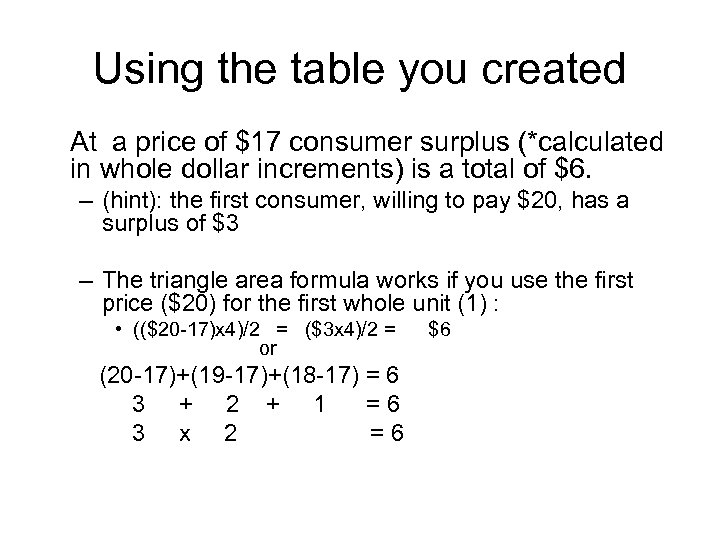Using the table you created At a price of \$17 consumer surplus (*calculated in whole dollar increments) is a total of \$6. – (hint): the first consumer, willing to pay \$20, has a surplus of \$3 – The triangle area formula works if you use the first price (\$20) for the first whole unit (1) : • ((\$20 -17)x 4)/2 = (\$3 x 4)/2 = or (20 -17)+(19 -17)+(18 -17) = 6 3 + 2 + 1 =6 3 x 2 =6 \$6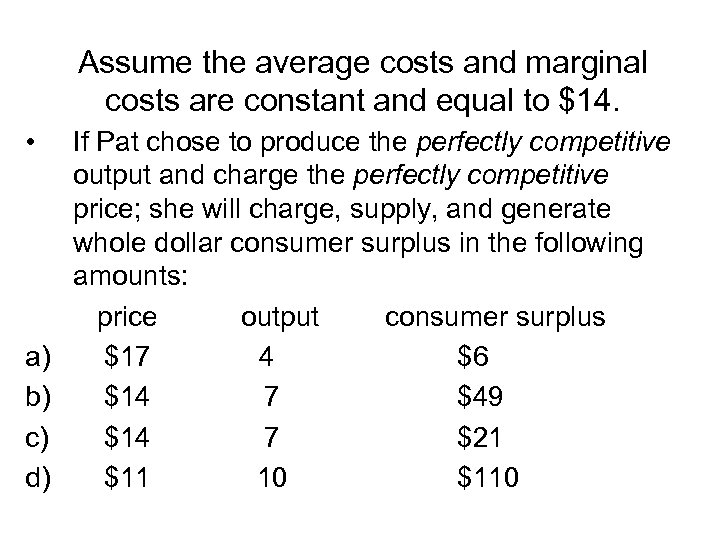Assume the average costs and marginal costs are constant and equal to \$14. • a) b) c) d) If Pat chose to produce the perfectly competitive output and charge the perfectly competitive price; she will charge, supply, and generate whole dollar consumer surplus in the following amounts: price output consumer surplus \$17 4 \$6 \$14 7 \$49 \$14 7 \$21 \$11 10 \$110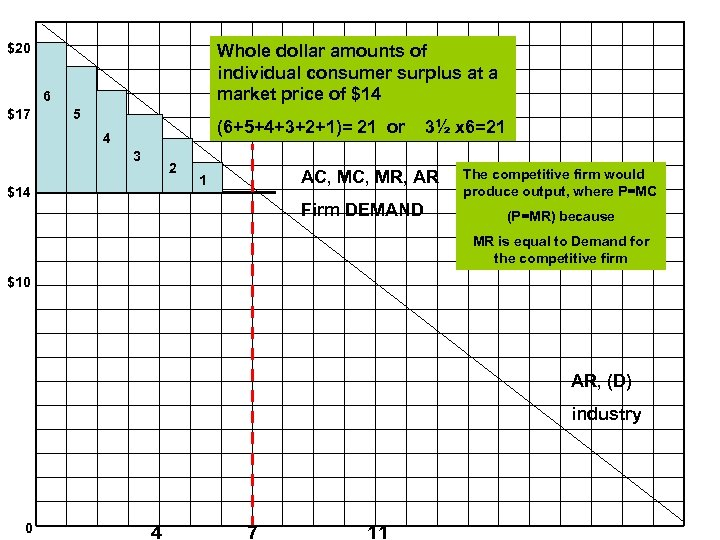\$20 Whole dollar amounts of individual consumer surplus at a market price of \$14 6 \$17 5 (6+5+4+3+2+1)= 21 or 4 3 2 \$14 3½ x 6=21 AC, MR, AR 1 Firm DEMAND The competitive firm would produce output, where P=MC (P=MR) because MR is equal to Demand for the competitive firm \$10 AR, (D) industry 0 4 7 11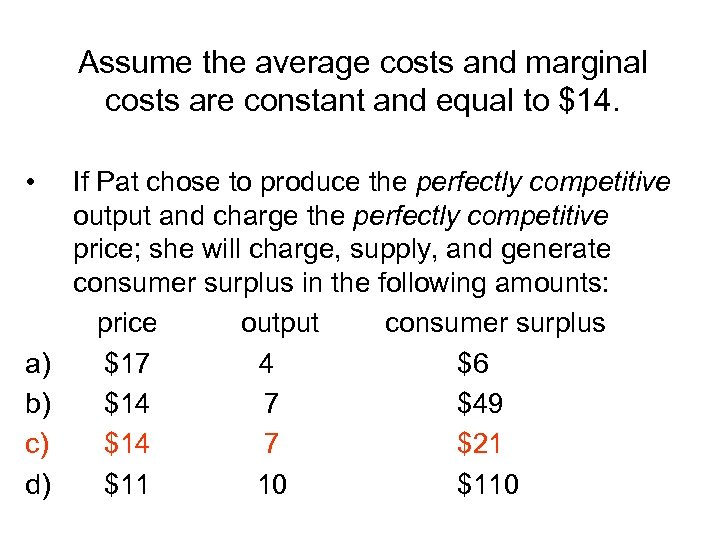Assume the average costs and marginal costs are constant and equal to \$14. • a) b) c) d) If Pat chose to produce the perfectly competitive output and charge the perfectly competitive price; she will charge, supply, and generate consumer surplus in the following amounts: price output consumer surplus \$17 4 \$6 \$14 7 \$49 \$14 7 \$21 \$11 10 \$110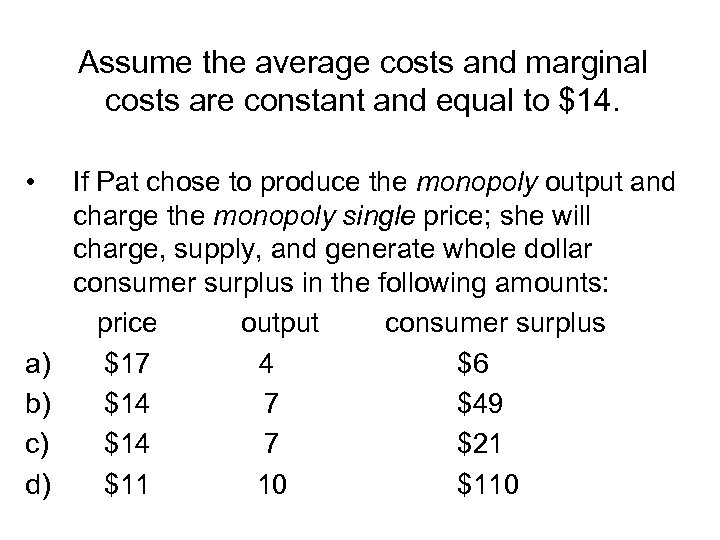Assume the average costs and marginal costs are constant and equal to \$14. • a) b) c) d) If Pat chose to produce the monopoly output and charge the monopoly single price; she will charge, supply, and generate whole dollar consumer surplus in the following amounts: price output consumer surplus \$17 4 \$6 \$14 7 \$49 \$14 7 \$21 \$11 10 \$110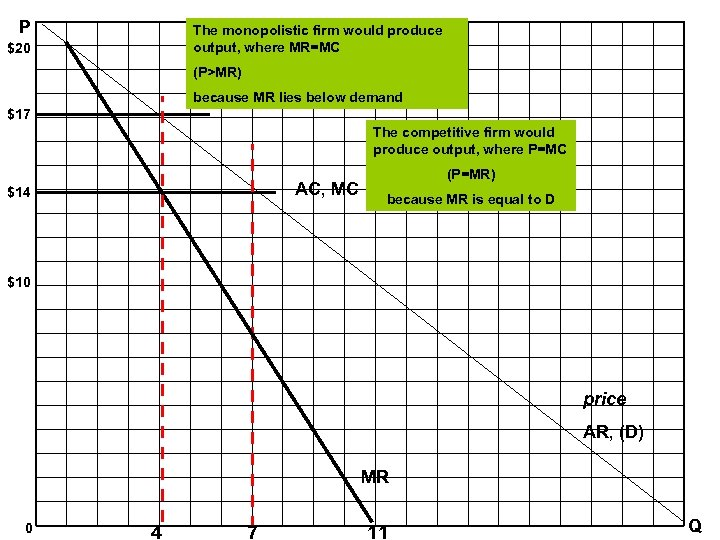P The monopolistic firm would produce output, where MR=MC \$20 (P>MR) because MR lies below demand \$17 The competitive firm would produce output, where P=MC AC, MC \$14 (P=MR) because MR is equal to D \$10 price AR, (D) MR 0 4 7 11 Q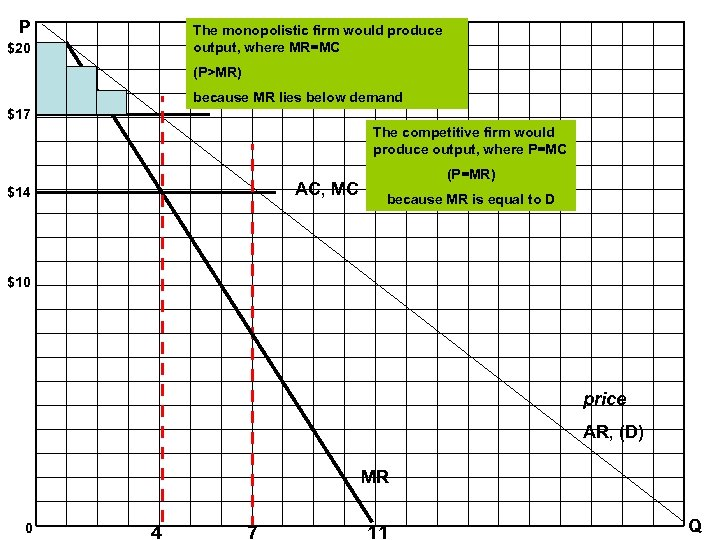P The monopolistic firm would produce output, where MR=MC \$20 (P>MR) \$17 because MR lies below demand 1 The competitive firm would produce output, where P=MC AC, MC \$14 (P=MR) because MR is equal to D \$10 price AR, (D) MR 0 4 7 11 Q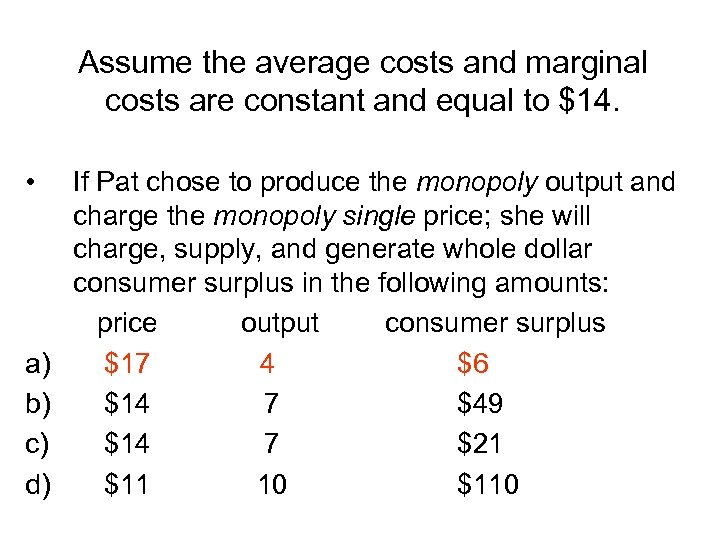Assume the average costs and marginal costs are constant and equal to \$14. • a) b) c) d) If Pat chose to produce the monopoly output and charge the monopoly single price; she will charge, supply, and generate whole dollar consumer surplus in the following amounts: price output consumer surplus \$17 4 \$6 \$14 7 \$49 \$14 7 \$21 \$11 10 \$110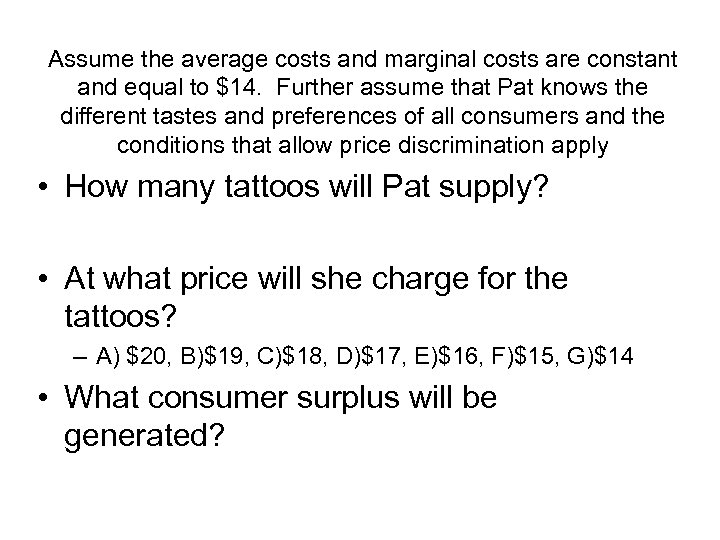Assume the average costs and marginal costs are constant and equal to \$14. Further assume that Pat knows the different tastes and preferences of all consumers and the conditions that allow price discrimination apply • How many tattoos will Pat supply? • At what price will she charge for the tattoos? – A) \$20, B)\$19, C)\$18, D)\$17, E)\$16, F)\$15, G)\$14 • What consumer surplus will be generated?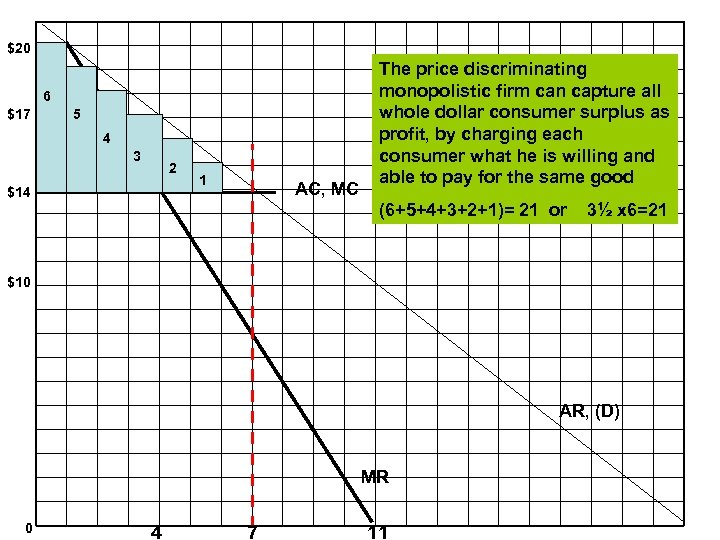\$20 6 \$17 5 4 3 2 \$14 1 AC, MC The price discriminating monopolistic firm can capture all whole dollar consumer surplus as profit, by charging each consumer what he is willing and able to pay for the same good (6+5+4+3+2+1)= 21 or 3½ x 6=21 \$10 AR, (D) MR 0 4 7 11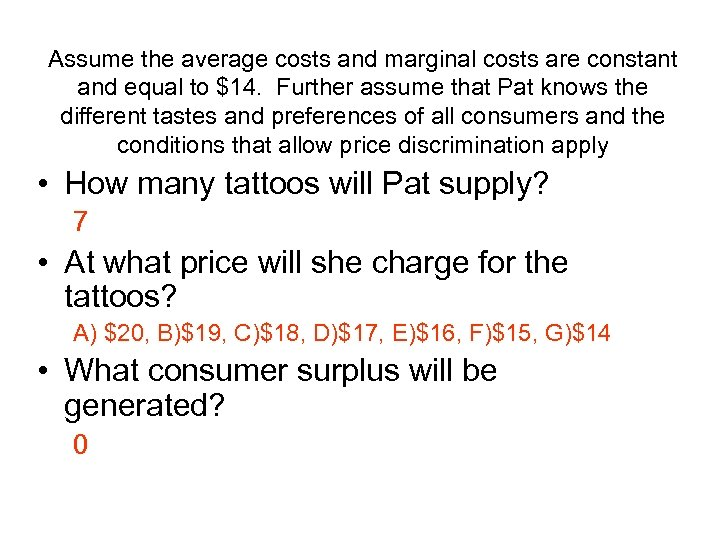Assume the average costs and marginal costs are constant and equal to \$14. Further assume that Pat knows the different tastes and preferences of all consumers and the conditions that allow price discrimination apply • How many tattoos will Pat supply? 7 • At what price will she charge for the tattoos? A) \$20, B)\$19, C)\$18, D)\$17, E)\$16, F)\$15, G)\$14 • What consumer surplus will be generated? 0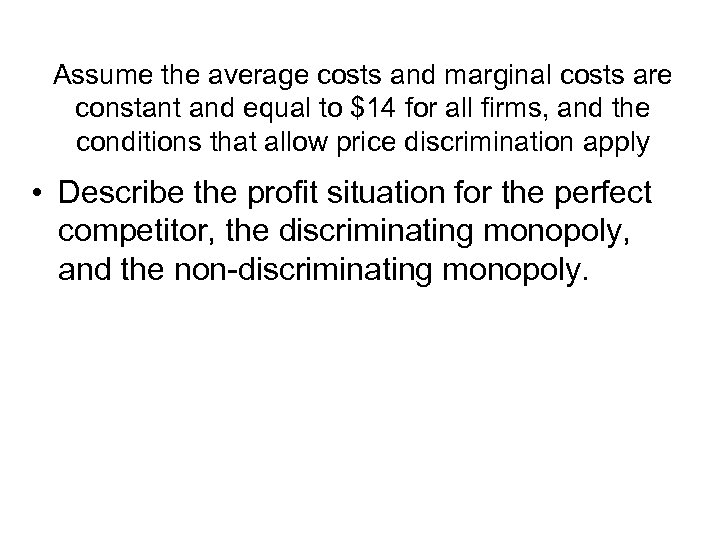Assume the average costs and marginal costs are constant and equal to \$14 for all firms, and the conditions that allow price discrimination apply • Describe the profit situation for the perfect competitor, the discriminating monopoly, and the non-discriminating monopoly.Assume the average costs and marginal costs are constant and equal to \$14 for all firms, and the conditions that allow price discrimination apply • Describe the profit situation for the perfect competitor, the discriminating monopoly, and the non-discriminating monopoly. • The profits for the discriminating monopoly will be highest, the perfect competitor will be lowest, and the non-discriminating monopoly will lie in between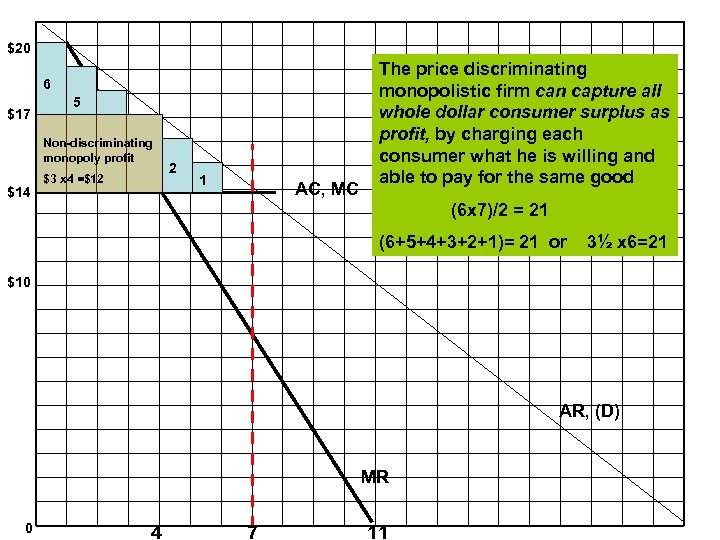\$20 6 \$17 5 Non-discriminating monopoly profit \$14 \$3 x 4 =\$12 2 1 AC, MC The price discriminating monopolistic firm can capture all whole dollar consumer surplus as profit, by charging each consumer what he is willing and able to pay for the same good (6 x 7)/2 = 21 (6+5+4+3+2+1)= 21 or 3½ x 6=21 \$10 AR, (D) MR 0 4 7 11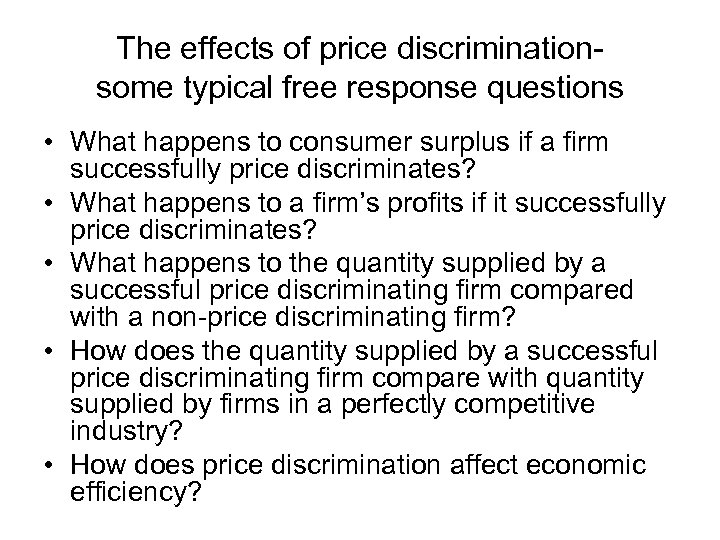The effects of price discriminationsome typical free response questions • What happens to consumer surplus if a firm successfully price discriminates? • What happens to a firm’s profits if it successfully price discriminates? • What happens to the quantity supplied by a successful price discriminating firm compared with a non-price discriminating firm? • How does the quantity supplied by a successful price discriminating firm compare with quantity supplied by firms in a perfectly competitive industry? • How does price discrimination affect economic efficiency?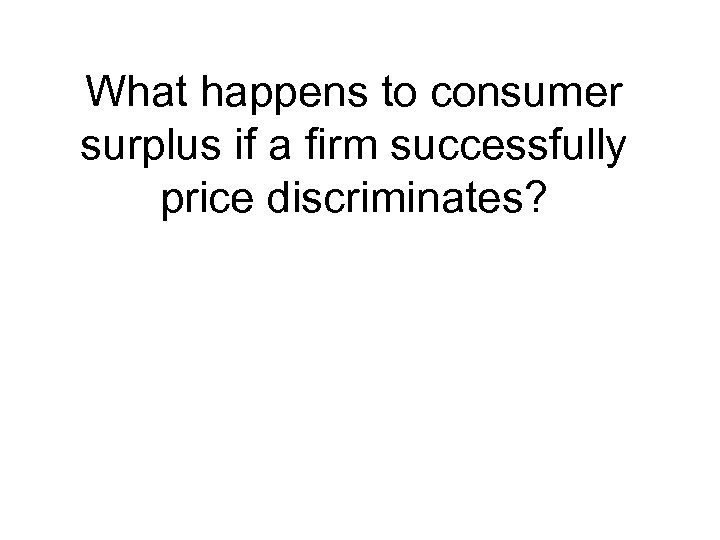What happens to consumer surplus if a firm successfully price discriminates?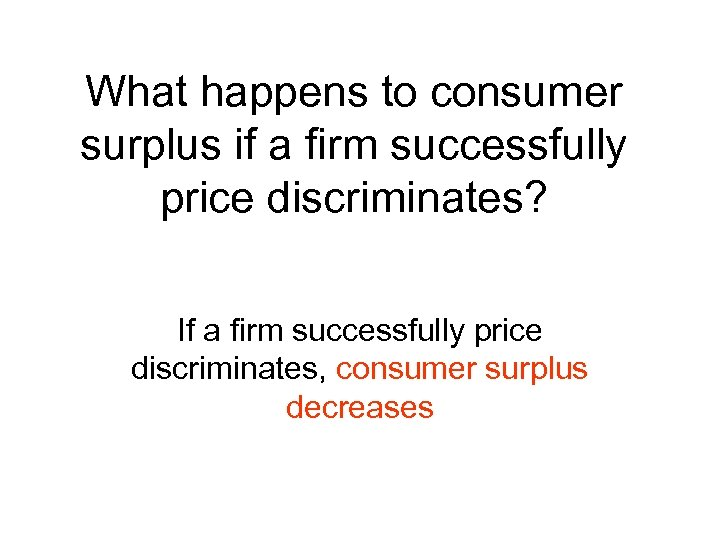What happens to consumer surplus if a firm successfully price discriminates? If a firm successfully price discriminates, consumer surplus decreases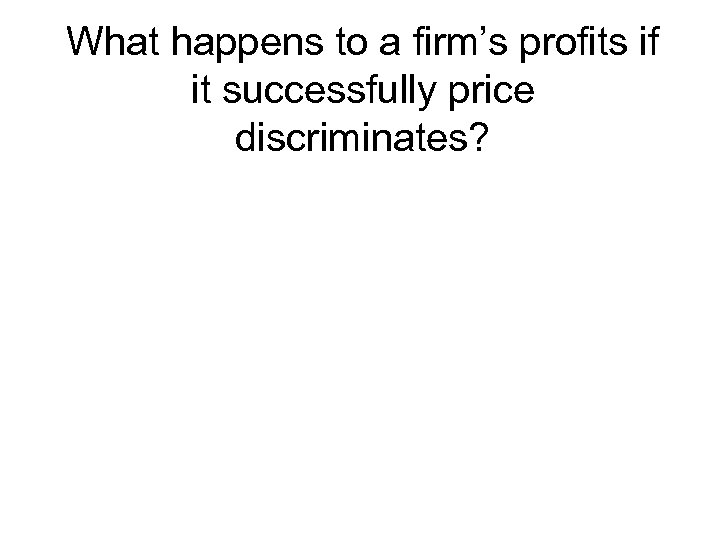What happens to a firm’s profits if it successfully price discriminates?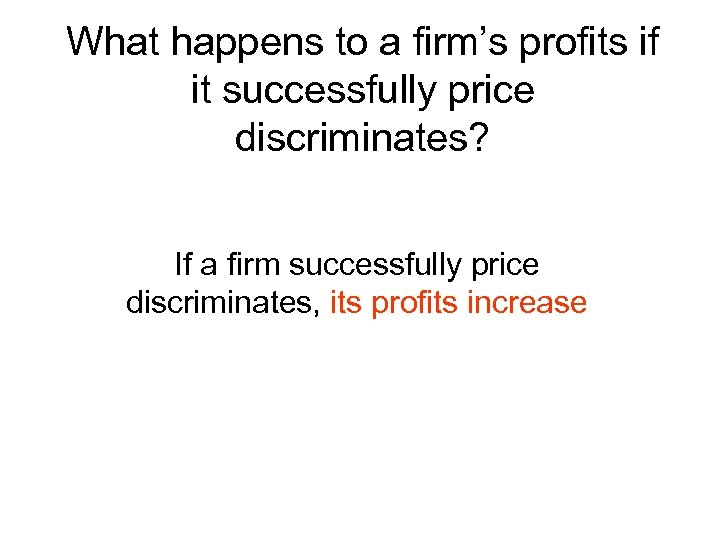What happens to a firm’s profits if it successfully price discriminates? If a firm successfully price discriminates, its profits increase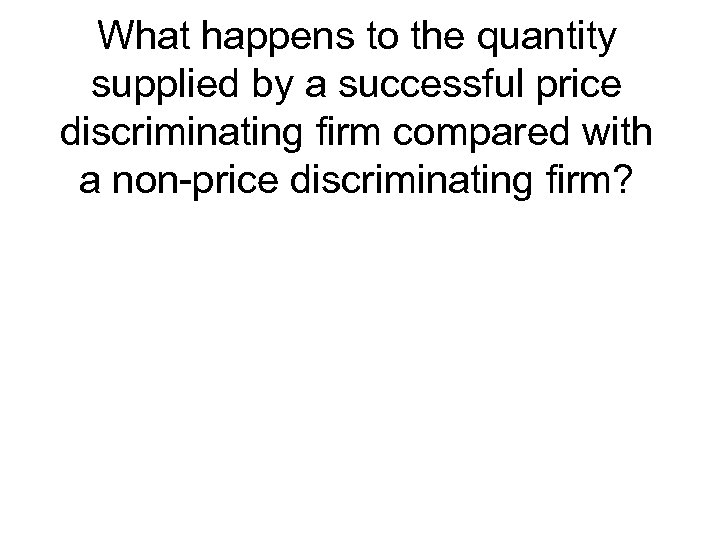What happens to the quantity supplied by a successful price discriminating firm compared with a non-price discriminating firm?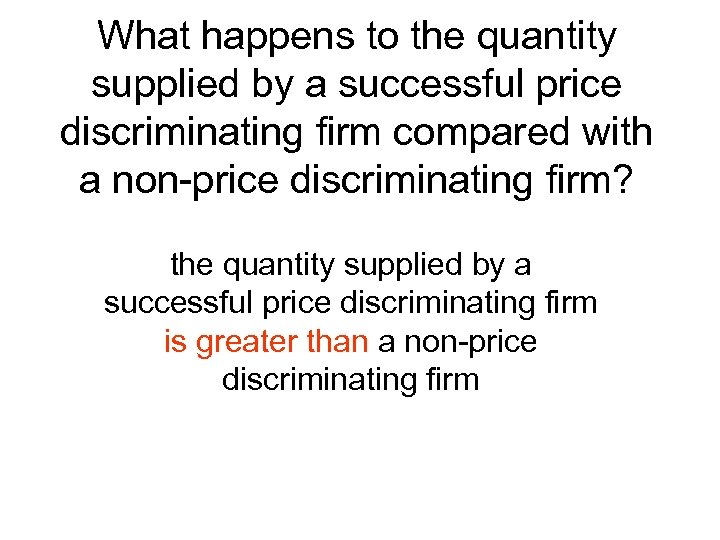What happens to the quantity supplied by a successful price discriminating firm compared with a non-price discriminating firm? the quantity supplied by a successful price discriminating firm is greater than a non-price discriminating firm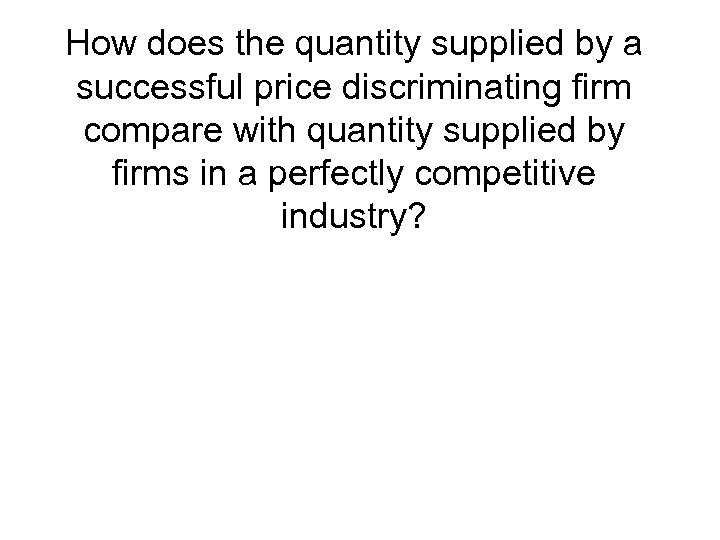How does the quantity supplied by a successful price discriminating firm compare with quantity supplied by firms in a perfectly competitive industry?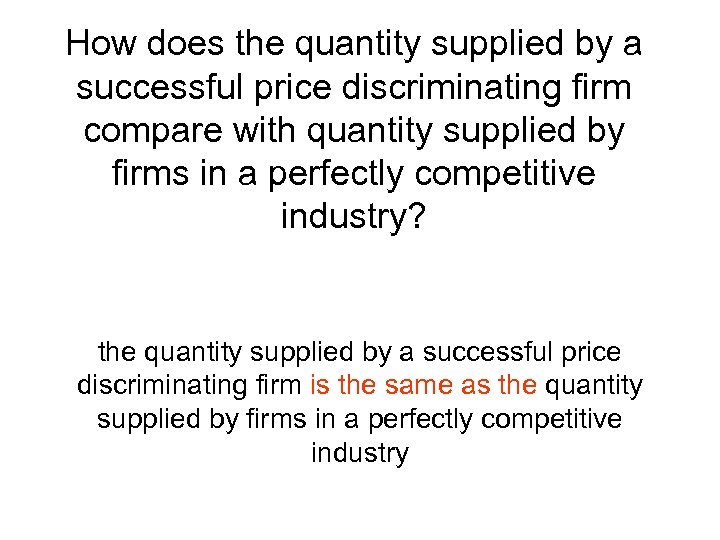How does the quantity supplied by a successful price discriminating firm compare with quantity supplied by firms in a perfectly competitive industry? the quantity supplied by a successful price discriminating firm is the same as the quantity supplied by firms in a perfectly competitive industry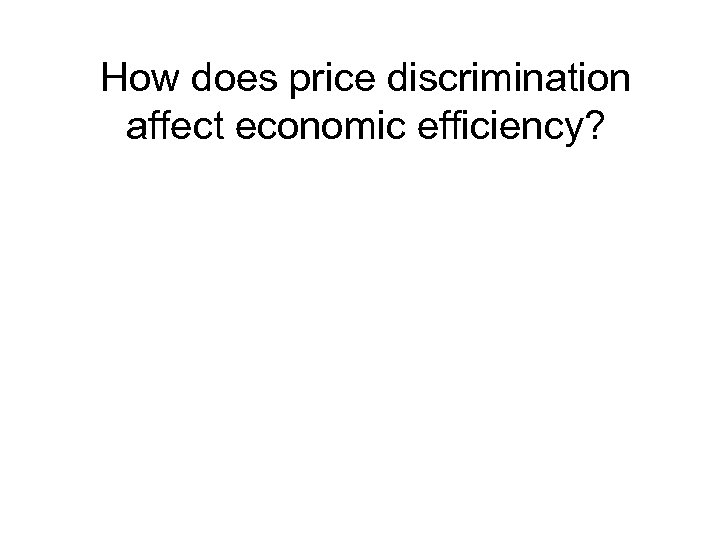How does price discrimination affect economic efficiency?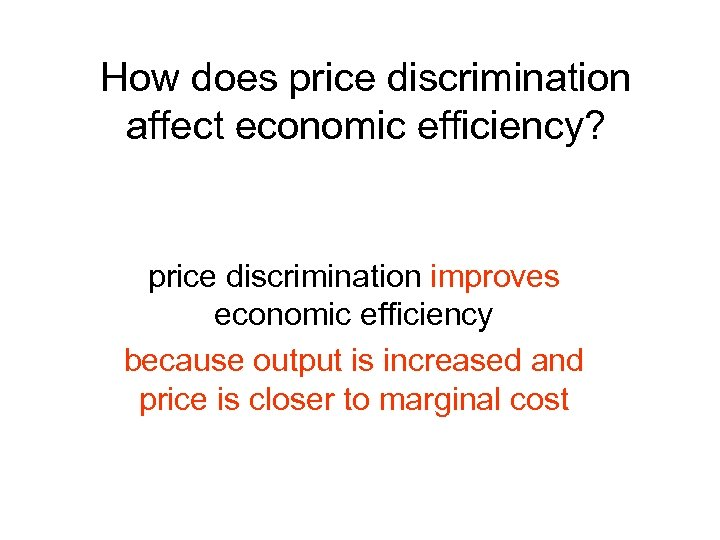How does price discrimination affect economic efficiency? price discrimination improves economic efficiency because output is increased and price is closer to marginal cost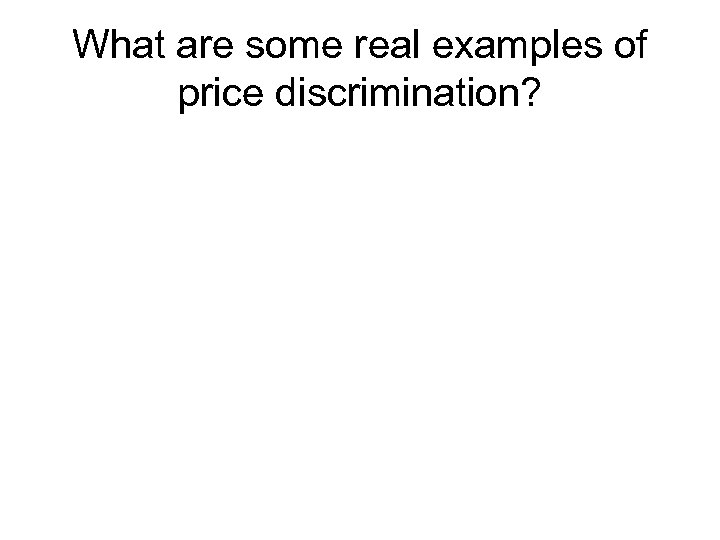What are some real examples of price discrimination?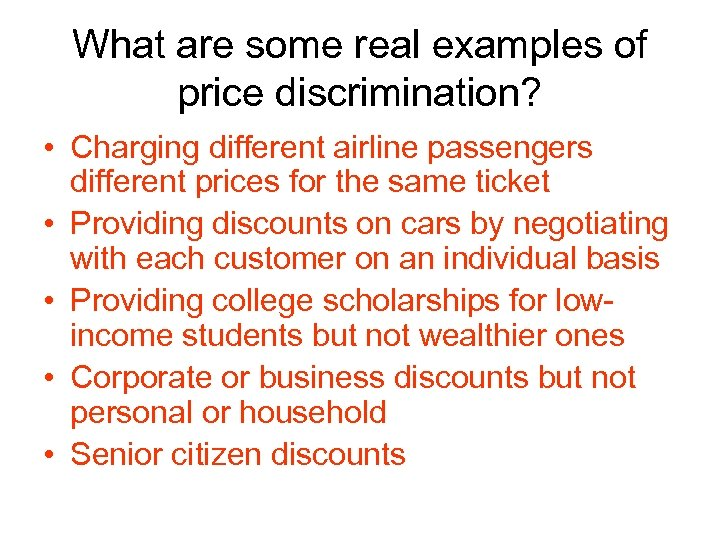What are some real examples of price discrimination? • Charging different airline passengers different prices for the same ticket • Providing discounts on cars by negotiating with each customer on an individual basis • Providing college scholarships for lowincome students but not wealthier ones • Corporate or business discounts but not personal or household • Senior citizen discounts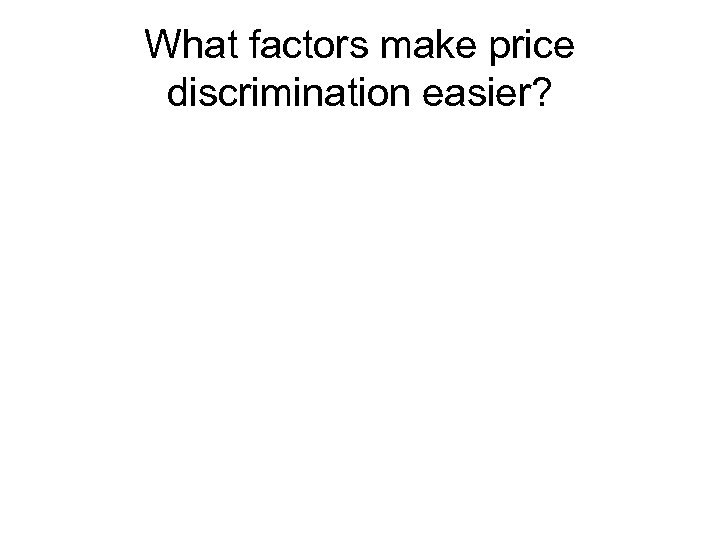What factors make price discrimination easier?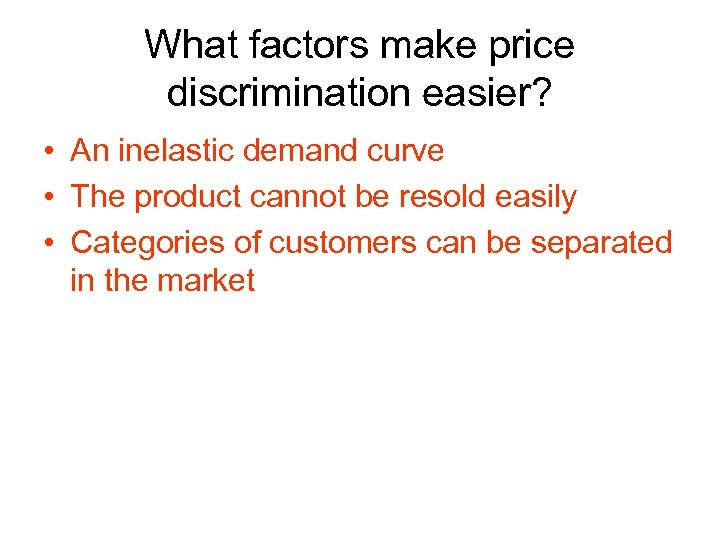What factors make price discrimination easier? • An inelastic demand curve • The product cannot be resold easily • Categories of customers can be separated in the market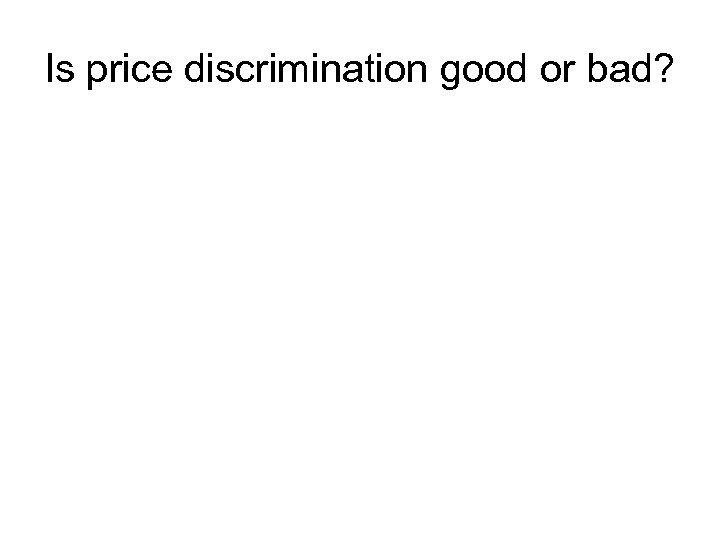Is price discrimination good or bad?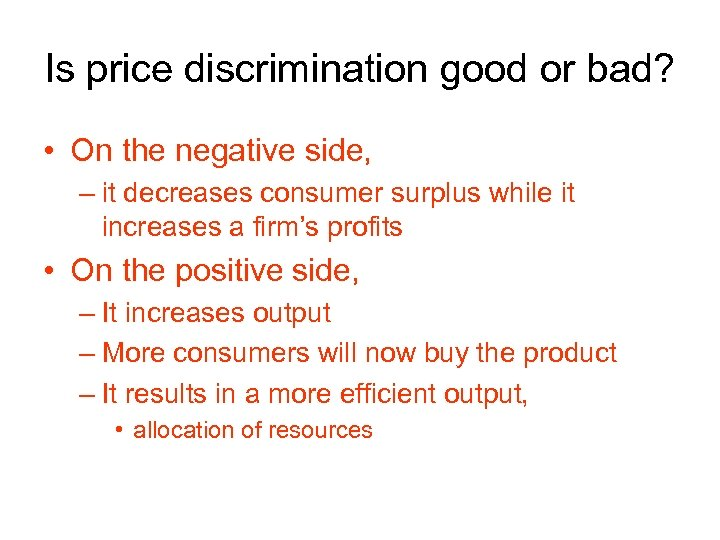Is price discrimination good or bad? • On the negative side, – it decreases consumer surplus while it increases a firm’s profits • On the positive side, – It increases output – More consumers will now buy the product – It results in a more efficient output, • allocation of resources